Views:

Every 3D compare will produce a set of statistics that can be found in the tabular view, properties, or report.  This article will explain what the values mean and how they are calculated.  The data used for the calculation can be accessed by exporting the deviation results of a 3D Compare entity.With sampling ratio set to 100% CX will calculate a deviation value for every vertex in the measured data.  The total number of points in the 3D compare will be called n.  Each measured vertex is defined by a Measured Pos. (Pm) and is associated with a Reference Pos. (Pr), which be defined by the Projection Direction (Shortest, Along Normal, Custom).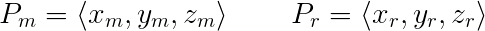For each measured point CX calculates a gap vector (GV), which is a vector that goes from the Pr to Pm.This gap vector is then converted to a scalar magnitude called the Gap Distance (D).  Gap distance is the deviation value at any given point.   If the measured point is on the negative side of the reference data the Gap Distance is given a negative value.  “Deviation” and “Gap Distance” can be used interchangeably.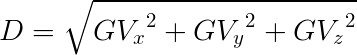Statistics:

Min: The smallest gap distance value.  Generally this is the largest negative deviation

Max : The largest gap distance value.  Generally this is the largest positive deviation

Average: The arithmetic mean of all the gap distances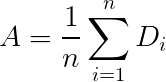RMS: The Root Mean Square which is a measure of the magnitude of all deviation values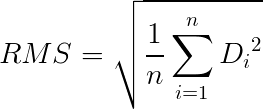Std. Dev.: The standard deviation of all the gap distance values.Var.Variance of the gap distance values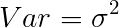+Avg: The mean of all the positive gap distances

-Avg: The mean of all the negative gap distances

In Tol.(%): The percentage of points that have a gap distance within the defined tolerance

Out  Tol.(%): The percentage of points that have a gap distance outside of the defined tolerance

Over Tol.(%): The percentage of points that are greater than the upper tolerance limit

Under Tol.(%): The percentage of points that are less than the upper tolerance limit